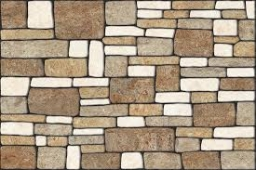# Performance 31371

According to the plan, seven pavers were to pave the square in 14 days. After two days of work, another 5 pavers came to help them. How many days from the arrival of the pavers will the work be completed? (tile performance is the same)

t =  9

### Step-by-step explanation:

7·14 = 2·7+(t-2)·(7+5)

12t = 108

t = 108/12 = 9

t = 9

Our simple equation calculator calculates it.Did you find an error or inaccuracy? Feel free to write us. Thank you!

Tips for related online calculators
Do you have a linear equation or system of equations and looking for its solution? Or do you have a quadratic equation?
Do you want to convert time units like minutes to seconds?